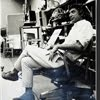## Eugene Ray SDSU 1970'sEugene Ray 1970's. photo credit: Tom Davis

## Friday, January 11, 2013

### RADIANT ARCHITECTURE VISION (ufo crash of 1800=light sphere/ ufo vision of 1947=>silver ship<) ^^^^^^^^^^^^^^^^^^^^^ LOOKING FOR THE INEFFABLE=> the search for TRANSCENDENCE a fabulous medium to NIRVANA ! ^^^^^^^^^^^^^^^^^^^^^ 2 D=3D=4D & beyond=ALCHEMY synergetic environs was a>WAY< ^^^^^^^^^^^^^^^^^^^^^ 5 PHOTOS PROVIDE REVELATION tulane effort to sdsu realizations>the search for nirvana<
>nola & san diego<
^^^^^^^^

+A L C H E M Y+
>did you notice the method of the<
+PROFESSOR+
>in florida building a time machine<
+WAS USING LIGHT+
>(>this was no surprise to our<
>efforts at tulane & sdsu<)
^^^^^^^^^^^^^

+INEFFABLE+
>search for nirvana in architecture<
>is a precious and sacred alchemy<
+COLOR=LIGHT=ENERGY+
>at its very heart<
^^^^^^^^

+TONIGHT'S PHOTOS+
^^^^^^^^^^
>the baton rouge 1800 ufo crash<
>was of a radiant sphere from<
>southwest to northeast<
^^^^^^^^^^^^
>the baton rouge 1947 sighting<
>was of a silver ship moving<
>from the west to east<
^^^^^^^^^^^

#1)            +"ARCHITECTURE+
>as situation, environment as light"<
>my first slide lecture explaining<
+SYNERGETIC+
+ENVIRONMENTS+
+1970+
>san diego state university<
^^^^^^^^^^^^^^

>the culmination of two years of<
>experimental work at tulane<
+BIOTRONICS+
>followed my early work<
>in the vieux carre<
^^^^^^^^^

#3b)                  >and<
>were the volumes research gave<
>as the most powerful resource<
^^^^^^^^^^^^^^^

#4)            +COLOR=LIGHT+
+ENERGY+
^^^^
>years ago in switzerland, near our<
>gruyere roots, doctors built a<
>terraces for all rooms<
^^^^^^^^^^^^

#5)         +DESIGN RESEARCH+
>provides<
+CONSTRUCTIONS+
>progendering exhibitions, lectures<
>and publications explaining the<
+ROOTS=ALCHEMY=MEDIA+
^^^^^^^^^^^^^^
+MEMORY+
>in my days of lectures abroad<
>it required a suitcase of ref<
+BOOKS & SLIDES+
^^^^^^^^^
+to present the entire<
+P O L E M I C+
^^^^^^^
>today the complex of this daily<
>web/blog presents this in<
+MULTI-DIMENSIONS+
>thanks to marianne, dave and<
>others of you out there who<
+KEEP ME GOING+
^^^^^^^^^

gene ray, lajolla, california

>eugene ray, mfa, architect<
>professor emeritus, sdsu<
^^^^^^^^^^^^^